# MCQs on Electrical Machines

##### Page 12 of 114. Go to page 1 2 3 4 5 6 7 8 9 10 11 12 13 14 15 16 17 18 19 20 21 22 23 24 25 26 27 28 29 30 31 32 33 34 35 36 37 38 39 40 41 42 43 44 45 46 47 48 49 50 51 52 53 54 55 56 57 58 59 60 61 62 63 64 65 66 67 68 69 70 71 72 73 74 75 76 77 78 79 80 81 82 83 84 85 86 87 88 89 90 91 92 93 94 95 96 97 98 99 100 101 102 103 104 105 106 107 108 109 110 111 112 113 114
01․ Which of the following motors is/are widely used?
squirrel cage induction motor
slip ring induction motor
either of these
none of the above

In slip ring induction motor brushes are present due to this frequent maintenance is required. Their design is complicated due to winding, slip rings and brushes and also expensive. Slip ring induction motor is comparatively having more weight than squirrel cage induction motor. The construction of squirrel cage induction motor is simple and robust and it is cheap as compared to slip ring induction motor. Therefore squirrel cage induction motor is widely used.

02․ A 4 pole 50 Hz induction motor is running at 1470 rpm. What is the slip value?
0.2
0.02
0.04
0.4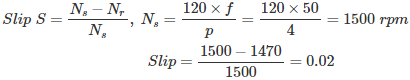03․ At stand still condition the value of slip is
1
0
infinite value
finite value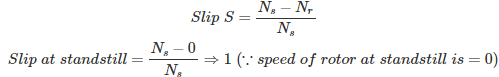04․ A 4 pole 50 Hz induction motor is running at 1470 rpm. The frequency of rotor current is
50 Hz
5 Hz
1 Hz
0 Hz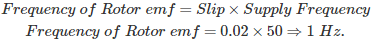05․ The speed of rotating magnetic field of 8 pole 50 Hz induction motor is
3000 rpm
1500 rpm
1000 rpm
750 rpm

The rotating magnetic field will rotate at synchronous speed.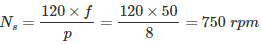Therefore the speed of rotating magnetic field is 750 rpm for 8 pole 50 Hz machine.

06․ When maximum starting torque is obtained, rotor power is
unity
zero
0.707 lagging
0.5 lagging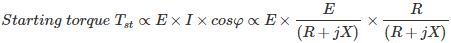Maximum starting torque will obtained when R = X i.e. the rotor resistance should exactly equal to the reactance at standstill. Under such condition rotor power factor is 0.707 lagging (presence of inductance).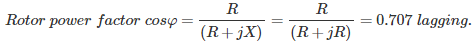07․ The condition for maximum running torque is
R = X
R = S.X
R = X/2
R = S.X/2

Maximum running torque occurs when S = R/X or R = S.X So the condition for maximum running torque is rotor resistance should be equal to slip times the rotor reactance at standstill.

08․ Which of the following will vary the maximum torque?
rotor resistance R
rotor reactance X
both 1 and 2
none of the above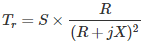The condition for maximum running torque is R = S.X By substituting the above value we can get the maximum torque. Tmax ∝ 1 / (2 X). Therefore the maximum torque will depend on the rotor reactance X only. The rotor resistance cannot vary the maximum torque developed in the motor but the slip or speed changes due to the rotor resistance.

09․ A motor operates in stable region at which of the following slip?
low slip region
high slip region
both 1 and 2
unity slip

The torque is proportional to slip only in the stable region and it is inversely proportional at higher operating slips. The entire operating region is divided into two regions. Running torque1.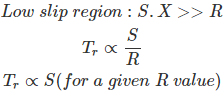Therefore under low slip region motor operates in stable region. 2.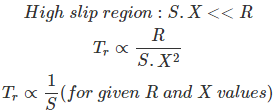Therefore under high slip regions motor operates in unstable region.

10․ In circle diagram for induction motor, diameter of circle represents which of the following ?
Slip.
Rotor current.
Running torque.
Line voltage.

Basically in the circle diagram for an induction motor, the diameter of the circle represents the rotor current. By using this circle, the current per phase with blocked rotor and full voltage on stator and the line current in amperes for any given load can be found out.

<<<1011121314>>>